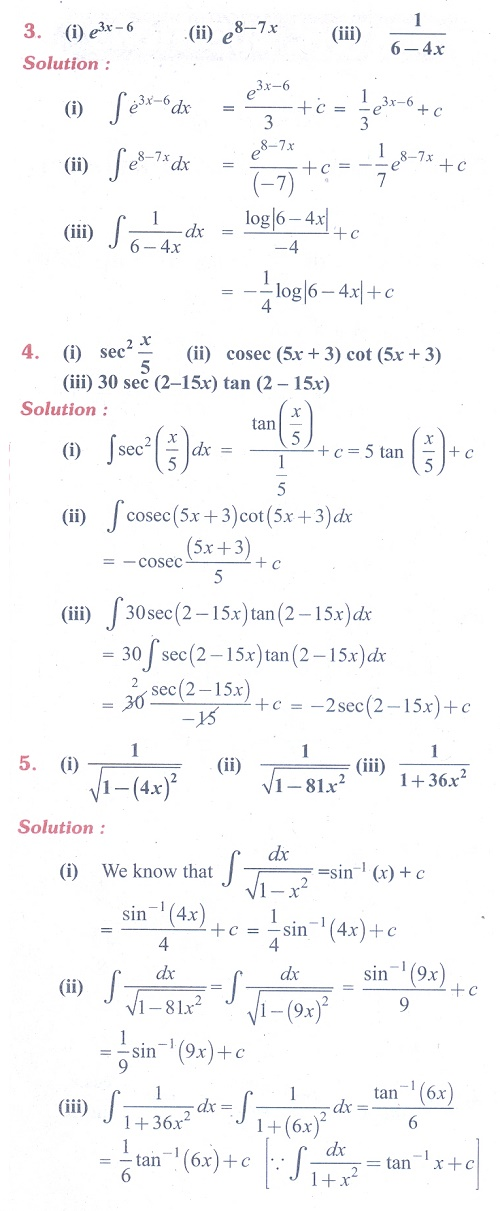Home | | Maths 11th std | Exercise 11.2: Integrals of the Form (ax + b)

# Exercise 11.2: Integrals of the Form (ax + b)

Maths Book back answers and solution for Exercise questions - Mathematics : Integral Calculus: Integrals of the Form (ax + b)Tags : Problem Questions with Answer, Solution | Mathematics , 11th Mathematics : UNIT 11 : Integral Calculus
Study Material, Lecturing Notes, Assignment, Reference, Wiki description explanation, brief detail
11th Mathematics : UNIT 11 : Integral Calculus : Exercise 11.2: Integrals of the Form (ax + b) | Problem Questions with Answer, Solution | Mathematics